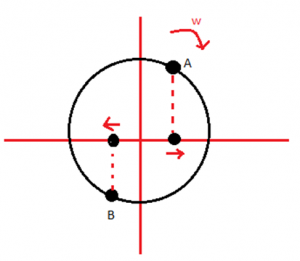# IIT JEE- Physics Study Techniques

While preparing for IIT JEE we face many complicated topics which we find very difficult to understand. In today’s time, we can learn physics online as there are many sites which provide different approaches with BYJU’S being the pioneers in this field. Another way to learn physics behind such topics is to refer to different books because different authors have different ways to visualize the problem and that can help us find an approach suitable for us. We have some simple IIT JEE Physics study techniques.

## IIT JEE- Physics Study Technique With Example

For example some people visualize SHM only as a trigonometric equation, x = A sin $omega$t, so a person having a problem with trigonometry will face the same problem here but if the same concept is  visualized as a motion on a circle and taking its projection on the x-axis as SHM might help.Once you are done with the topics you must solve different type of problems because it is one of the best ways to learn physics. Also, they should be practised at regular intervals through daily practice problems which can have a set of 10 questions covering the topics you have completed in the past. This helps in revising the concepts in an efficient way. Also when we are initially learning a topic we should try to solve problems with two or more approaches that are different from one another, because it helps in developing a deeper understanding of the topic. And we begin to realize under which situation we should take which approach. For example problems on mechanics can be solved by both forces as well as energy method. We should try solving the initial problems by both methods then only we can choose which approach is better depending on the requirement of the question. Students will always search how to learn physics easily but actually, there is no easy way to learn physics. Only a dedicated approach along with practice can help us learn physics.

To know more about simple approaches to complicated concepts join BYJU’S.